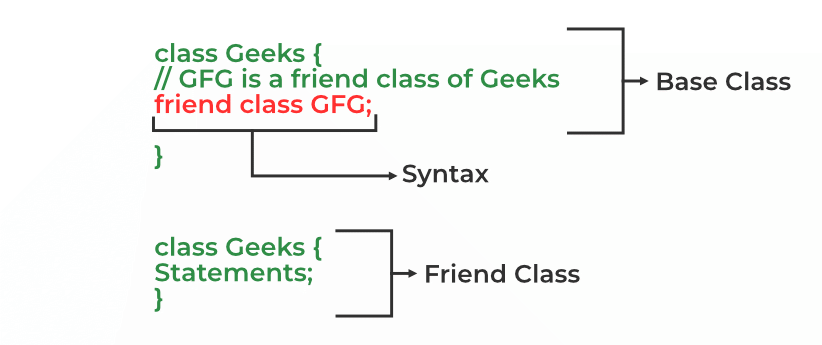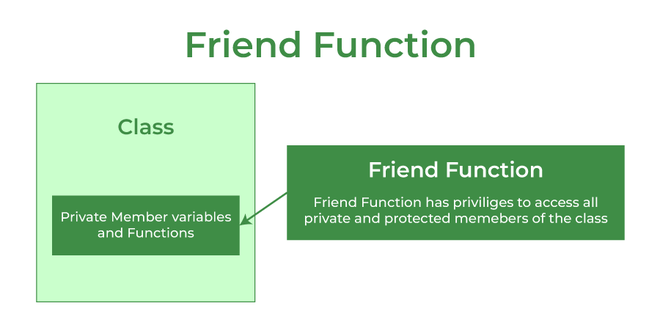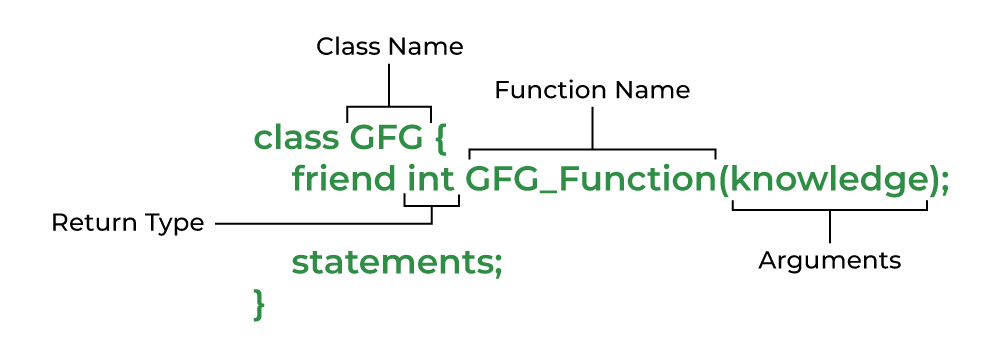Open in App
Not now

# Friend Class and Function in C++

• Difficulty Level : Medium
• Last Updated : 06 Jan, 2023

A friend class can access private and protected members of other classes in which it is declared as a friend. It is sometimes useful to allow a particular class to access private and protected members of other classes. For example, a LinkedList class may be allowed to access private members of Node.

We can declare a friend class in C++ by using the friend keyword.

Syntax:

`friend class class_name;    // declared in the base class`Friend Class Syntax

Example:

## C++

 `// C++ Program to demonstrate the``// fucntioning of a friend class``#include ``using` `namespace` `std;`` ` `class` `GFG {``private``:``    ``int` `private_variable;`` ` `protected``:``    ``int` `protected_variable;`` ` `public``:``    ``GFG()``    ``{``        ``private_variable = 10;``        ``protected_variable = 99;``    ``}`` ` `    ``// friend class declaration``    ``friend` `class` `F;``};`` ` `// Here, class F is declared as a``// friend inside class GFG. Therefore,``// F is a friend of class GFG. Class F``// can access the private members of``// class GFG.``class` `F {``public``:``    ``void` `display(GFG& t)``    ``{``        ``cout << ``"The value of Private Variable = "``             ``<< t.private_variable << endl;``        ``cout << ``"The value of Protected Variable = "``             ``<< t.protected_variable;``    ``}``};`` ` `// Driver code``int` `main()``{``    ``GFG g;``    ``F fri;``    ``fri.display(g);``    ``return` `0;``}`

Output

```The value of Private Variable = 10
The value of Protected Variable = 99```

Note: We can declare friend class or function anywhere in the base class body whether its private, protected or public block. It works all the same.

## Friend Function

Like a friend class, a friend function can be granted special access to private and protected members of a class in C++. They are the non-member functions that can access and manipulate the private and protected members of the class for they are declared as friends.

A friend function can be:

1. A global function
2. A member function of another classFriend Function in C++

Syntax:

```friend return_type function_name (arguments);    // for a global function
or
friend return_type class_name::function_name (arguments);    // for a member function of another class```Friend Function Syntax

### 1. Global Function as Friend Function

We can declare any global function as a friend function. The following example demonstrates how to declare a global function as a friend function in C++:

Example:

## C++

 `// C++ program to create a global function as a friend``// function of some class``#include ``using` `namespace` `std;`` ` `class` `base {``private``:``    ``int` `private_variable;`` ` `protected``:``    ``int` `protected_variable;`` ` `public``:``    ``base()``    ``{``        ``private_variable = 10;``        ``protected_variable = 99;``    ``}``     ` `      ``// friend function declaration``    ``friend` `void` `friendFunction(base& obj);``};`` ` ` ` `// friend function definition``void` `friendFunction(base& obj)``{``    ``cout << ``"Private Variable: "` `<< obj.private_variable``         ``<< endl;``    ``cout << ``"Protected Variable: "` `<< obj.protected_variable;``}`` ` `// driver code``int` `main()``{``    ``base object1;``    ``friendFunction(object1);`` ` `    ``return` `0;``}`

Output

```Private Variable: 10
Protected Variable: 99```

In the above example, we have used a global function as a friend function. In the next example, we will use a member function of another class as a friend function.

### 2. Member Function of Another Class as Friend Function

We can also declare a member function of another class as a friend function in C++. The following example demonstrates how to use a member function of another class as a friend function in C++:

Example:

## C++

 `// C++ program to create a member function of another class``// as a friend function``#include ``using` `namespace` `std;`` ` `class` `base; ``// forward definition needed``// another class in which function is declared``class` `anotherClass {``public``:``    ``void` `memberFunction(base& obj);``};`` ` `// base class for which friend is declared``class` `base {``private``:``    ``int` `private_variable;`` ` `protected``:``    ``int` `protected_variable;`` ` `public``:``    ``base()``    ``{``        ``private_variable = 10;``        ``protected_variable = 99;``    ``}`` ` `    ``// friend function declaration``    ``friend` `void` `anotherClass::memberFunction(base&);``};`` ` `// friend function definition``void` `anotherClass::memberFunction(base& obj)``{``    ``cout << ``"Private Variable: "` `<< obj.private_variable``         ``<< endl;``    ``cout << ``"Protected Variable: "` `<< obj.protected_variable;``}`` ` `// driver code``int` `main()``{``    ``base object1;``    ``anotherClass object2;``    ``object2.memberFunction(object1);`` ` `    ``return` `0;``}`

Output

```Private Variable: 10
Protected Variable: 99```

Note: The order in which we define the friend function of another class is important and should be taken care of. We always have to define both the classes before the function definition. Thats why we have used out of class member function definition.

### Features of Friend Functions

•  A friend function is a special function in C++ that in spite of not being a member function of a class has the privilege to access the private and protected data of a class.
• A friend function is a non-member function or ordinary function of a class, which is declared as a friend using the keyword “friend” inside the class. By declaring a function as a friend, all the access permissions are given to the function.
• The keyword “friend” is placed only in the function declaration of the friend function and not in the function definition or call.
• A friend function is called like an ordinary function. It cannot be called using the object name and dot operator. However, it may accept the object as an argument whose value it wants to access.
• A friend function can be declared in any section of the class i.e. public or private or protected.

Below are some more examples of friend functions in different scenarios:

## C++

 `// C++ Program to demonstrate ``// how friend functions work as``// a bridge between the classes``#include ``using` `namespace` `std;`` ` `// Forward declaration``class` `ABC; `` ` `class` `XYZ {``    ``int` `x;`` ` `public``:``    ``void` `set_data(``int` `a) ``    ``{ ``      ``x = a; ``    ``}`` ` `    ``friend` `void` `max(XYZ, ABC);``};`` ` `class` `ABC {``    ``int` `y;`` ` `public``:``    ``void` `set_data(``int` `a) ``    ``{ ``      ``y = a; ``    ``}`` ` `    ``friend` `void` `max(XYZ, ABC);``};`` ` `void` `max(XYZ t1, ABC t2)``{``    ``if` `(t1.x > t2.y)``        ``cout << t1.x;``    ``else``        ``cout << t2.y;``}`` ` `// Driver code``int` `main()``{``    ``ABC _abc;``    ``XYZ _xyz;``    ``_xyz.set_data(20);``    ``_abc.set_data(35);`` ` `    ``// calling friend function``    ``max(_xyz, _abc); ``    ``return` `0;``}`

Output

`35`

The friend function provides us with a way to access private data but it also has its demerits. Following is the list of advantages and disadvantages of friend functions in C++:

• A friend function is able to access members without the need of inheriting the class.
• The friend function acts as a bridge between two classes by accessing their private data.
• It can be used to increase the versatility of overloaded operators.
• It can be declared either in the public or private or protected part of the class.

• Friend functions have access to private members of a class from outside the class which violates the law of data hiding.
• Friend functions cannot do any run-time polymorphism in their members.

## Important Points About Friend Functions and Classes

1. Friends should be used only for limited purposes. Too many functions or external classes are declared as friends of a class with protected or private data access lessens the value of encapsulation of separate classes in object-oriented programming.
2. Friendship is not mutual. If class A is a friend of B, then B doesn’t become a friend of A automatically.
3. Friendship is not inherited. (See this for more details)
4. The concept of friends is not in Java.

My Personal Notes arrow_drop_up